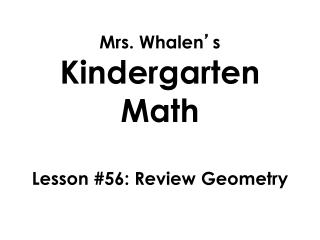DownloadDownload PresentationMrs. Whalen ’ s Kindergarten Math Lesson #56: Review Geometry

# Mrs. Whalen ’ s Kindergarten Math Lesson #56: Review Geometry

Download Presentation## Mrs. Whalen ’ s Kindergarten Math Lesson #56: Review Geometry

- - - - - - - - - - - - - - - - - - - - - - - - - - - E N D - - - - - - - - - - - - - - - - - - - - - - - - - - -
##### Presentation Transcript

1. Mrs. Whalen’s Kindergarten Math Lesson #56: Review Geometry

2. All of these shapes are flat shapes. They can not stand up. If I cut them out they could not stand up because they are not solid shapes. rectangle square triangle hexagon circle

3. We call these shapes 2-dimensional shapes or 2-D shapes.

4. Let’s watch! https://www.youtube.com/watch?v=htcuPenTg38

5. I see a squareabove a circle.

6. I see a circle below a triangle.

7. I see a triangle beside a rectangle.

8. I see a triangle in front of a hexagon. A hexagon has 5 sides and 5 corners. A triangle has 3 sides and 3 corners.

9. I see a hexagon behind a triangle.

10. I see a hexagon next to a square.

11. A square has 4 sides and 4 corners. side corner

12. These two triangles are the same. They have the same number of sides and same number of corners.

13. These two shapes are different. A circle has no corners and a rectangle has 4 corners. corner

14. Some shapes are solid. The shapes can stack on top of each other, slide around or roll. They are 3-dimensional shapes or 3-D shapes.

15. Solid Shapes

16. Let’s watch! https://www.youtube.com/watch?v=K9L9l86N-xM

17. Both of these shapes are closed figures. A closed figure is where all of the sides connect.

18. Here is an example of an open and closed figure.

19. The triangle and square are closed. The sides that make up these shapes meet to form a corner. corner corner

20. This is a closed circle. This is an open circle.

21. Let’s look at a cube and a square.

22. Both the cube and the square are made up of straight lines and are closed figures.

23. The cube is made up of 6 squares.

24. Let’s try making a square with 2 triangles.

25. By moving the triangles we can make a square.

26. By moving the triangles we can make a square.

27. Let’s move triangles over to see how many small triangles make a large triangle.

28. Let’s move triangles over to see how many small triangles make a large triangle. 1 2 3

29. Let’s move triangles over to see how many small triangles make a large triangle. 1 4 5 2 6 3

30. For the last 3 spaces we have to turn the triangles upside down. 1 4 5 2 6 3

31. For the last 3 spaces we have to turn the triangles upside down. 1 • 7 4 5 7 2 8 6 9 3

32. It took 9 small triangles to fill up the large triangle. 1 • 7 4 5 7 2 8 6 9 3

33. Let’s make a house together. You help me. You draw a house using shapes on your paper with me. First let’s start with a square.

34. Next draw a triangle on top. This will be the roof.

35. Next draw a rectangle in the middle of the bottom of the square. This will be the door.

36. We made a house out of shapes!

37. Good Job!!

38. Goodbye! See you next time!